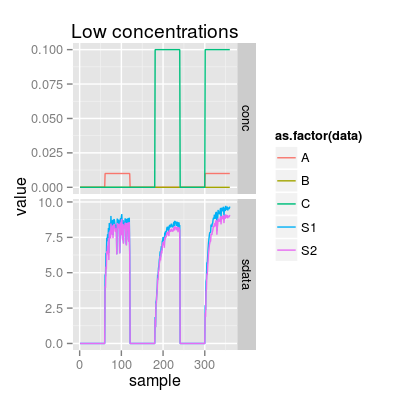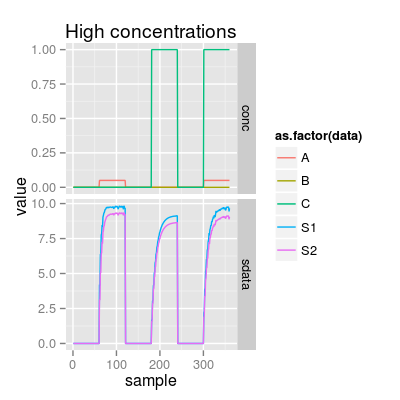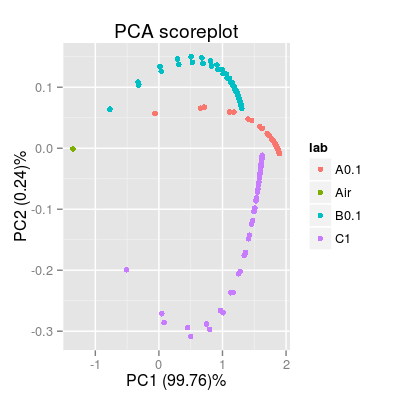Method nconst.

Usage

defaultParSensorDynamics()

SensorDynamics(...)

Arguments

...
parameters of constructor.

Value

List of the default parameters.

Description

Method nconst.

Method trange.

Method tconst.

Class SensorDynamics emulates a temporal dynamics of the sensor as a low-pass filter.

Function to get default constructor parameters of class SensorDynamics.

Constructor method of SensorDynamics Class.

Wrapper function SensorDynamics.

Details

Slots of the class:

 num Sensor number (1:17). The default value is 1. gases Gas indices. ngases The number of gases. gnames Names of gases. concUnits Concentration units external to the model. concUnitsInt Concentration units internal to the model. tunit Time length of the gas pulses. The default value is 60. enableDyn A logical value indicates whether time dynamics is enabled. It is TRUE if tunit is greater than one. tconst Time constants of the low pass filter.

Methods of the class:

 predict Predicts a temporal response of the sensor. coef Extracts the time constants of the filter.

The plot method has one type (parameter y):

 predict Depicts a temporal signal of the model.

Examples

### Sensor dynamics: default initialization sdyn <- SensorDynamics() sdyn # equivalent to: show(sdyn)
Sensor Dynamics Model (num 1)
print(sdyn)
Sensor Dynamics Model - num 1 - 3 gases A, B, C
#plot(sdyn) ### SensorDynamics as a part of SensorModel sm <- SensorModel(tunit = 60) sdyn <- as(sm, "SensorDynamics") ### Demo 1: response to pulses of different concentrations set.seed(5) sa <- SensorArray(tunit = 60, csd = 1, ssd = 0, dsd = 0) # mixture at low concentrations p1 <- plotSignal(sa, set = c("A 0.01", "C 0.1", "A 0.01, C 0.1"), main = "Low concentrations") p1# mixture at high concentrations p2 <- plotSignal(sa, set = c("A 0.05", "C 1", "A 0.05, C 1"), main = "High concentrations") p2### sa <- SensorArray(tunit = 60, nsd = 0) p3 <- plotSignal(sa, set = c("A", "B", "C")) p3p4 <- plotPCA(sa, set = c("A", "B", "C")) p4